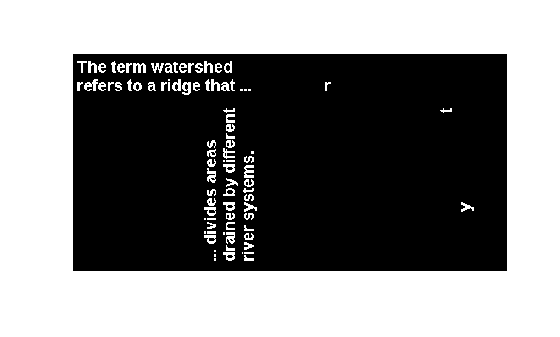Documentation

### This is machine translation

Mouseover text to see original. Click the button below to return to the English version of the page.

# bwselect

Select objects in binary image

## Syntax

``BW2 = bwselect(BW,c,r,n)``
``BW2 = bwselect(BW,n)``
``````[BW2,idx] = bwselect(___)``````
``BW2 = bwselect(x,y,BW,xi,yi,n)``
``````[x,y,BW2,idx,xi,yi] = bwselect(___)``````

## Description

example

````BW2 = bwselect(BW,c,r,n)` returns a binary image containing the objects that overlap the pixel (`r,c`), where `n` specifies the connectivity. Objects are connected sets of `on` pixels, that is, pixels having a value of `1`. By default, `bwselect` looks for 4-connected objects.```
````BW2 = bwselect(BW,n)` displays the image `BW` on the screen and lets you select the (`r,c`) coordinates using the mouse. If you omit `BW`, `bwselect` operates on the image in the current axes. Use normal button clicks to add points. Press Backspace or Delete to remove the previously selected point. A shift-click, right-click, or double-click selects the final point; press Return to finish the selection without adding a point.```
``````[BW2,idx] = bwselect(___)``` returns the linear indices of the pixels belonging to the selected objects.```
````BW2 = bwselect(x,y,BW,xi,yi,n)` uses the vectors `x` and `y` to establish a nondefault spatial coordinate system for `BW`. The arguments `xi` and `yi` are scalars or equal-length vectors that specify locations in this coordinate system.```
``````[x,y,BW2,idx,xi,yi] = bwselect(___)``` returns the `XData` and `YData` in `x` and `y`, the output image in `BW2`, linear indices of all the pixels belonging to the selected objects in `idx`, and the specified spatial coordinates in `xi` and `yi`.```

## Examples

collapse all

Select objects in a binary image and create a new image containing only those objects.

Read binary image into the workspace.

`BW = imread('text.png');`

Specify the locations of objects in the image using row and column indices.

```c = [43 185 212]; r = [38 68 181];```

Create a new binary image containing only the selected objects. This example specifies 4-connected objects.

`BW2 = bwselect(BW,c,r,4);`

Display the original image and the new image side-by-side.

`imshowpair(BW,BW2,'montage');`## Input Arguments

collapse all

Input binary image, specified as a 2-D, nonsparse, logical or numeric matrix. If you do not specify an output argument, `bwselect` displays the output image in a new figure.

Example: `BW = imread('text.png');`

Data Types: `single` | `double` | `int8` | `int16` | `int32` | `int64` | `uint8` | `uint16` | `uint32` | `uint64` | `logical`

Column index, specified as a numeric scalar or vector. If `c` and `r` are equal-length vectors, `BW2` contains the sets of objects overlapping with any of the pixels `(r(k),c(k))`.

Example: `c = [43 185 212];`

Data Types: `single` | `double` | `int8` | `int16` | `int32` | `int64` | `uint8` | `uint16` | `uint32` | `uint64`

Row index, specified as a numeric scalar or vector. If `r` and `c` are equal-length vectors, `BW2` contains the sets of objects overlapping with any of the pixels `(r(k),c(k))`.

Example: `r = [38 68 181];`

Data Types: `single` | `double` | `int8` | `int16` | `int32` | `int64` | `uint8` | `uint16` | `uint32` | `uint64`

Connectivity, specified as either the value `4` or `8`.

ValueDescription
`4`4-connected objects
`8`8-connected objects

Example: `BW2 = bwselect(BW,c,r,4);`

Data Types: `single` | `double` | `int8` | `int16` | `int32` | `int64` | `uint8` | `uint16` | `uint32` | `uint64`

x coordinates of nondefault coordinate system, specified as a numeric scalar or vector.

Example: `x = [19.5 23.5];`

Data Types: `single` | `double` | `int8` | `int16` | `int32` | `int64` | `uint8` | `uint16` | `uint32` | `uint64`

y coordinates of nondefault coordinate system, specified as a numeric scalar or vector.

Example: `y = [8.0 12.0];`

Data Types: `single` | `double` | `int8` | `int16` | `int32` | `int64` | `uint8` | `uint16` | `uint32` | `uint64`

x coordinates of locations in nondefault coordinate system, specified as a numeric scalar or vector.

Example: `x = [19.5 23.5];`

Data Types: `single` | `double` | `int8` | `int16` | `int32` | `int64` | `uint8` | `uint16` | `uint32` | `uint64`

y coordinates of locations in nondefault coordinate system, specified as a numeric scalar or vector.

Example: `y = [8.0 12.0];`

Data Types: `single` | `double` | `int8` | `int16` | `int32` | `int64` | `uint8` | `uint16` | `uint32` | `uint64`

## Output Arguments

collapse all

Binary image containing objects that overlap the specified pixels, returned as a logical array.

If you do not specify an output argument, `bwselect` displays the output image in a new figure.

Linear indices of the pixels belonging to the selected objects, returned as a numeric vector.